# (c) (6 points) Show a formal proof that the height of a Red-Black Tree h with...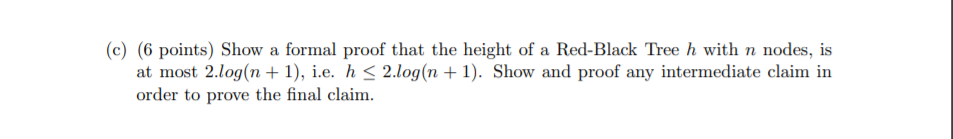(c) (6 points) Show a formal proof that the height of a Red-Black Tree h with n nodes, is at most 2.log(n + 1), i.e. h < 2.log(n + 1). Show and proof any intermediate claim in order to prove the final claim.

Solution

A red black tree has the following properties:

1) Every node has a color either red or black.

2) Root of tree is always black.

3) A red node cannot have a red parent or red child.

4) Every path from a node to any of its descendant (NULL node) has the same number of black nodes.

For a binary tree, let k be the minimum number of nodes from root to a leaf node, then total number of nodes in the binary tree >=2k - 1

Nomber of nodes at root level (Level 1) = 1

Number of nodes in level 2 = 2

Number of nodes in level 2 = 22

Number of nodes in level 2 = 23

Number of nodes in level 2 = 24

Number of nodes in level 2 = 25

..................................................

Number of nodes in level k = 2k-1

So, minimmum number of nodes in binary tree = 1 + 2 + 22 + 23 + 24 + .................+ 2k-1

= 1.(2k - 1) / 2 - 1 = 2k - 1

n >= 2k​​​​​​​ - 1

n+1>=2k​​​​​​​

2k​​​​​​​ <= n+1

log22k = log (n+1)

k = log(n+1)

So a root to leaf path will have at-most log2(n+1) black nodes.

Since a red node cannot have a red parent or a red child, at least 1/2 of the nodes on any path from root to an external node are black. Since the longest path of the tree is h, the black-height of the root must be at least h/2

So for a Red-Black Tree of height h has black-height >= h/2.

h/2 <=   log2(n+1)

h <= 2.log2(n+1)

So the height of red black tree with n nodes is atmost 2.log2(n+1)

#### Earn Coin

Coins can be redeemed for fabulous gifts.

Similar Homework Help Questions
• ### Show that the tree height of a height-balanced binary search tree with n nodes is O(log...

Show that the tree height of a height-balanced binary search tree with n nodes is O(log n). (Hint: Let T(h) denote the fewest number of nodes that a height-balanced binary search tree of height h can have. Express T(h) in terms of T(h-1) and T(h-2). Then, find a lower bound of T(h) in terms of T(h-2). Finally, express the lower bound of T(h) in terms of h.)

• ### Select statements that are true about Red Black Tree (there may be more than one correct...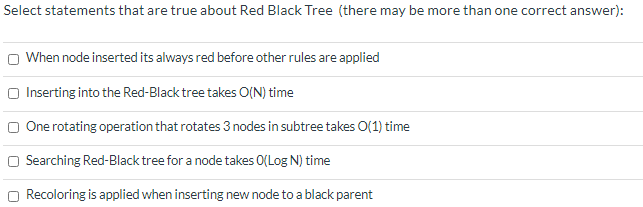Select statements that are true about Red Black Tree (there may be more than one correct answer): When node inserted its always red before other rules are applied Inserting into the Red-Black tree takes O(N) time One rotating operation that rotates 3 nodes in subtree takes O(1) time Searching Red-Black tree for a node takes O(Log N) time Recoloring is applied when inserting new node to a black parent

• ### Num #2 Below, you are given a correct Red-Black tree, in which red nodes are shown...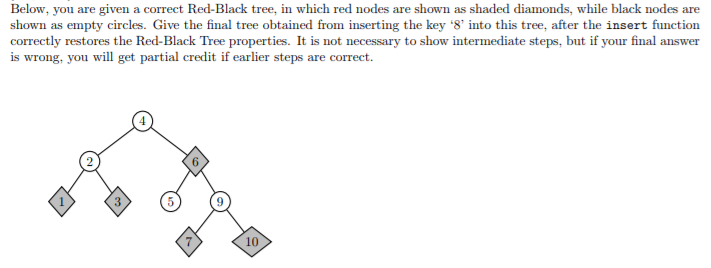Num #2 Below, you are given a correct Red-Black tree, in which red nodes are shown as shaded diamonds, while black nodes are shown as empty circles. Give the final tree obtained from inserting the key '8 into this tree, after the insert function correctly restores the Red-Black Tree properties. It is not necessary to show intermediate steps, but if your final answer is wrong, you will get partial credit if earlier steps are correct. 4 (6 3 10

• ### Java Red-Black Tree Assignment Part 3: Red-black trees Left-leaning red-black trees, as they are described in...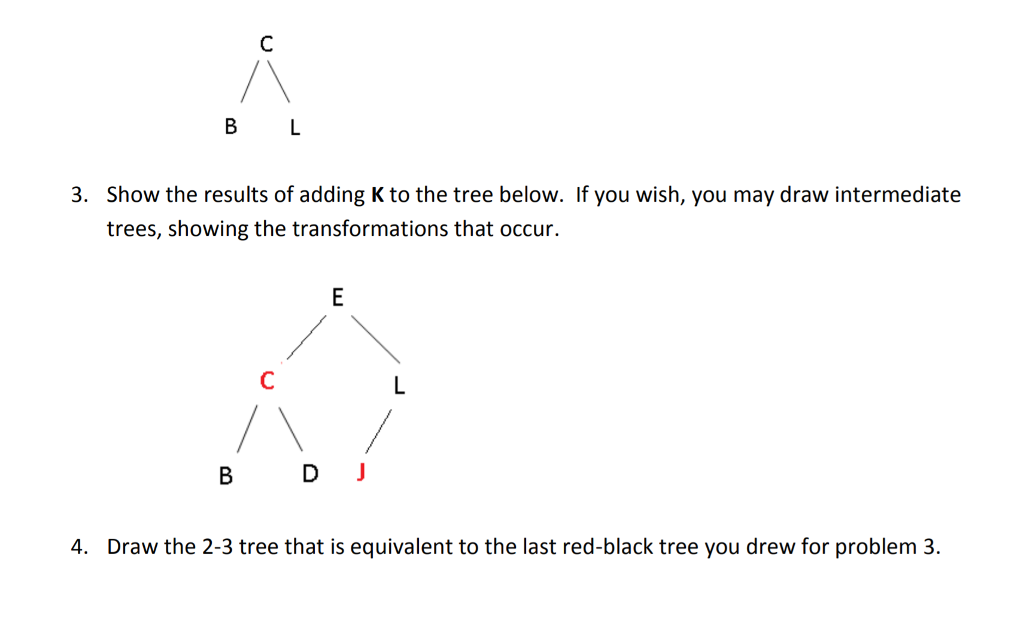Java Red-Black Tree Assignment Part 3: Red-black trees Left-leaning red-black trees, as they are described in the Sedgewick and Wayne text, undergo 3 possible transformations as they grow: rotateLeft, rotateRight, and flipColors. You may refer to the text or my lecture notes for the details of each of these tree transformations. In red-black tree diagrams, you will see 2 ways to represent color. In the first, edges are colored, either red or black. In the other version, color information is...

• ### red-black tree C++ or C

1. You should implement a data structure for tree node with data eld of type int; and coloreld of type char, taking values of either r (for red)or b (for black). It should also have the fields for parent, lchild and rchild.2. You should implement a function named RBwrite to write out the current red-black tree tothe screen, where the tree is stored in a pre-orderformat.3. You should implement a function named RBread to read in a red-black tree from...

• ### Prove this Lemma. Lemma 2.2 A binary tree with height h has at most 2h+1-1 nodes....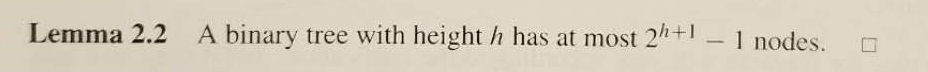Prove this Lemma. Lemma 2.2 A binary tree with height h has at most 2h+1-1 nodes. □

• ### Refer to the definition of Full Binary Tree from the notes. For a Full Binary Tree...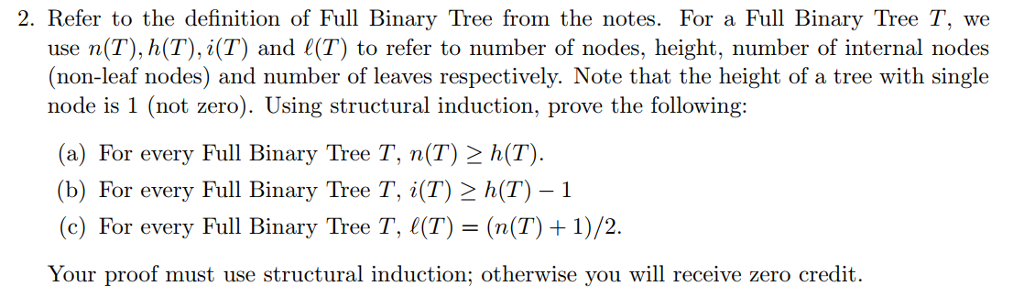Refer to the definition of Full Binary Tree from the notes. For a Full Binary Tree T, we use n(T), h(T), i(T) and l(T) to refer to number of nodes, height, number of internal nodes (non-leaf nodes) and number of leaves respectively. Note that the height of a tree with single node is 1 (not zero). Using structural induction, prove the following: (a) For every Full Binary Tree T, n(T) greaterthanorequalto h(T). (b) For every Full Binary Tree T, i(T)...

• ### A binary search tree includes n nodes and has an height h. Check all that applies...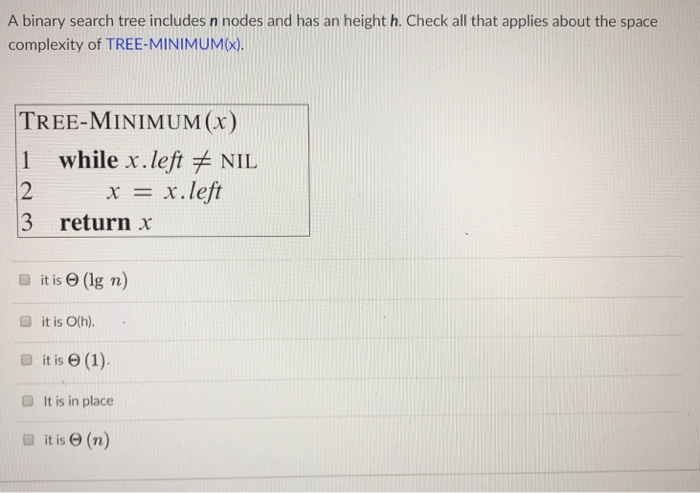A binary search tree includes n nodes and has an height h. Check all that applies about the space complexity of TREE-MINIMUMX) TREE-MINIMUM () 1 while x. left NIL 2 3 return x x x.left O it is e (lg n) ■ it is 0(h). D it is e (1) ■ It is in place ■ it is Θ (n) A binary search tree includes n nodes and has an height h. Check all that applies about the space complexity...

• ### Data structures c++ 1- What is the search time in an AVL tree with n nodes....

Data structures c++ 1- What is the search time in an AVL tree with n nodes. Select one or more: a. O(2^n) b. O(height * log n) c. O(log n) d. O(height) e. O(log height) f. O(n) g. O(1) h. O(2^height)

• ### Question 46 2 pts Which of the following is incorrect about a red-black tree? Group of...

Question 46 2 pts Which of the following is incorrect about a red-black tree? Group of answer choices a. The leaves are always red b. A red-black tree is equivalent to a 2-4 tree c. A red node must have a black parent d. Every path from the root to the leaves must have the same number of red nodes e. a and d Flag this Question Question 47 2 pts Given the following maxheap, assuming heap entries start at...

Free Homework App